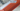# Reverse String - Leet Code Solution

September 04, 2020## Problem Statement

Write a function that reverses a string. The input string is given as an array of characters char[].

Do not allocate extra space for another array, you must do this by modifying the input array in-place with O(1) extra memory.

You may assume all the characters consist of printable ascii characters.

Example

``````Input: ["h","e","l","l","o"]
Output: ["o","l","l","e","h"]``````

## Solution

Its very simple problem to solve.

• Take two index variables
• Start one from left, other from extreme end
• Start swapping their values, and move them
Left in forward, and extreme right in backward
• Repeat above untill both index variables cross each other

## Code

``````public void reverseString(char[] s) {
int left = 0;
int end = s.length-1;
while (left < end) {
//swap
char temp = s[left];
s[left] = s[end];
s[end] = temp;

left ++;
end --;
}
}``````

## Complexity

Its `O(n)`